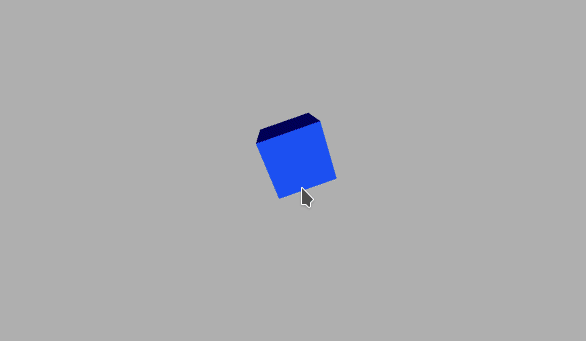Open in App
Not now

# p5.js perspective() Method

• Last Updated : 04 Mar, 2021

The perspective() function in p5.js is used to define a perspective projection for the camera in a 3D sketch.

Objects that are close to the camera appear in their real size while those that are further away from the camera appear smaller than the real size. This is known as foreshortening. The parameters of this function define a viewing volume with the shape of the truncated pyramid. The field of view, aspect ratio, near and far clipping planes can be set using the given parameters.

Syntax:

`perspective([fovy], [aspect], [near], [far])`

Parameters: This function accept four parameters as mentioned above and described below:

• fovy: This is a number that defines the camera frustum vertical field of view seen from the bottom to the top. This is an optional parameter.
• aspect: This is a number that defines the camera frustum aspect ratio. This is an optional parameter.
• near: This is a number that defines the frustum near plane length. This is an optional parameter.
• far: This is a number that defines the frustum far plane length. This is an optional parameter.

The below example illustrates the perspective() function in p5.js:

Example:

## Javascript

 `function` `setup() { ` `  ``createCanvas(600, 400, WEBGL); ` `} ` ` `  `function` `draw() { ` `  ``background(175); ` `   `  `  ``// Map the fov to the mouse x-axis ` `  ``let fov = map(mouseX, 0, width, 0, PI); ` `  ``let cZ = (height/2.0) / tan((PI/3)/2.0); ` `   `  `  ``// Set the perspective to the fov ` `  ``perspective(fov, float(width)/float(height), ` `              ``cZ/10.0, cZ*10.0); ` `   `  `  ``ambientLight(255); ` `   `  `  ``rotateZ(frameCount * 0.02); ` `  ``rotateX(frameCount * 0.02); ` ` `  `  ``noStroke(); ` `  ``normalMaterial(); ` `  ``box(100, 100, 100); ` `   `  `}`

Output:Reference:https://p5js.org/reference/#/p5/perspective

My Personal Notes arrow_drop_up
Related Articles